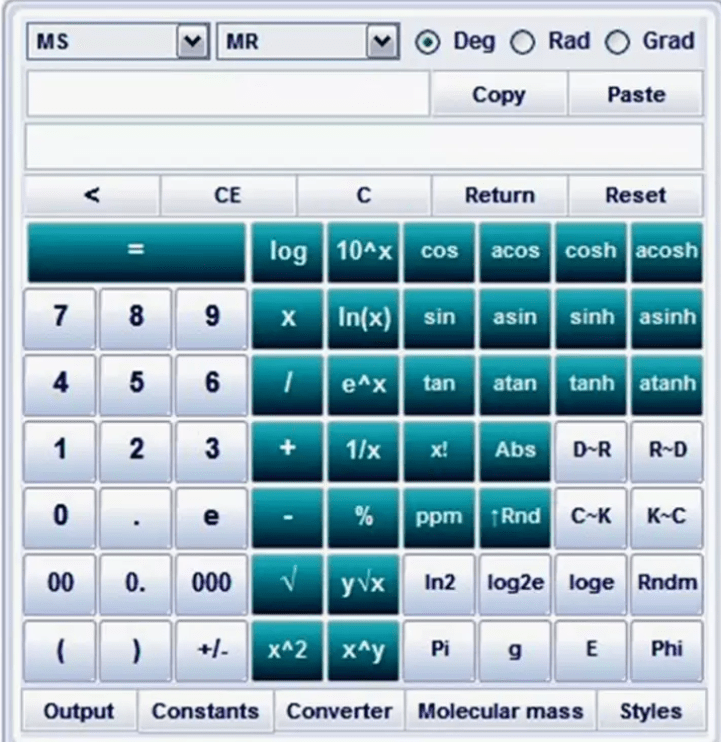# Python LibrariesPosted by

This is in continuation of Learn Python with Jupyter Notebook .

I discussed about data structure techniques Dictionary and Set. Understanding the difference between all data structure techniques. I also discussed about different installation options and overview of Python world.

### Key Takeaway

Today I will further compare Python control and loop commands with C. I will discuss working of Jupyter notebook and  will provide brief introduction  of Python core libraries using the same.

### Control Statements

#### if condition

• ##### In C,  the general format is

if (testExpression1)

{ // statement(s) }

else if(testExpression2)

{ // statement(s) }

else if (testExpression 3)

{ // statement(s) }

………………………

else

{ // statement(s) }

• ##### In Python,  the general format is

if testExpression1 :

// statement(s)

elif testExpression2:

// statement(s)

elif testExpression3:

// statement(s)

……………………..

else :

// statement(s)

#### for loop

##### In C, the general format is

for (initialize; check_Condition; Modify)

{ // statement }

##### In Python, the general format is

for val in sequence:

//statements

Ex: for x in range (1,5) :

y – x*2

#### While

##### In C, the general format is

while(condition) {

//statement(s);

}

##### In Python, the general format is

while testExpression1 :

// statement(s)

### Python Core Libraries :

SciPy  is  a Python based open-source software  ecosystem for mathematics, science, and engineering. It has following core packages :

• NumPy  N-dimensional array package(BSD License) . It is used in scientific computations where large multi-dimensions arrays,matrices and high level mathematic functions are used.
• SciPy Library  (License) utilizes NumPy fast array manipulation . It provides efficient routines for integration and optimization.
•  (). It is Python 2D plotting library to  generate publication quality figures. Here is one example to draw Dolphins. One can refer to Artist API for detail. Matplotlib was originally written as a Python alternative for MATLAB users, and much of its syntax reflects that fact.
• SymPy   (BSD License) is  Python library for symbolic mathematics. It aims to become a full-featured computer algebra system (CAS). Here is an example of syntax tree used in CAS for (a+b)*c  where * is root(Parent) and (a+b),C are operands(children)• Pandas  (BSD License) provides data structure and data analysis tools.
• IPython  (BSD License) is interactive shell and supports data visualization

#### Anaconda Navigator here.

JupyterLab is an interactive development environment for working with notebooks, code and data. However Jupyter notebook is recommended for beginners.

conda install -c anaconda anaconda-navigator

I take  Jupyter notebook analogy  as the modern ADVANCED Software  Scientific Calculator.  Lets  check this in notebook itself.